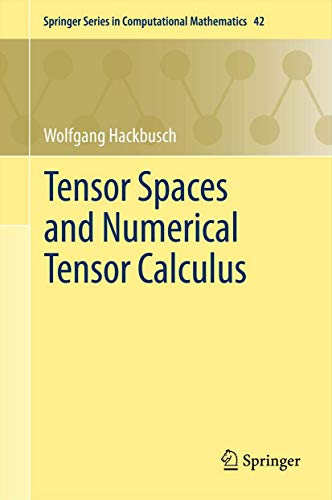# Tensor Spaces and Numerical Tensor Calculus (Springer Series in Computational Mathematics)

## Hackbusch, Wolfgang

4 durchschnittliche Bewertung
( 1 Bewertungen bei Goodreads )
)Alle Exemplare der Ausgabe mit dieser ISBN anzeigen:

SPECIAL NUMERICAL TECHNIQUES ARE ALREADY NEEDED TO DEAL WITH NXN MATRICES FOR LARGE N.TENSOR DATA ARE OF SIZE NXNX...XN=N^D, WHERE N^D EXCEEDS THE COMPUTER MEMORY BY FAR. THEY APPEAR FOR PROBLEMS OF HIGH SPATIAL DIMENSIONS. SINCE STANDARD METHODS FAIL, A PARTICULAR TENSOR CALCULUS IS NEEDED TO TREAT SUCH PROBLEMS. THE MONOGRAPH DESCRIBES THE METHODS HOW TENSORS CAN BE PRACTICALLY TREATED AND HOW NUMERICAL OPERATIONS CAN BE PERFORMED. APPLICATIONS ARE PROBLEMS FROM QUANTUM CHEMISTRY, APPROXIMATION OF MULTIVARIATE FUNCTIONS, SOLUTION OF PDE, E.G., WITH STOCHASTIC COEFFICIENTS, ETC. ?

Die Inhaltsangabe kann sich auf eine andere Ausgabe dieses Titels beziehen.

Cr�ticas:

“The present book Tensor spaces and numerical tensor calculus by Wolfgang Hackbusch to a large extent fills the gap between theory on finite-dimensional tensor spaces and tensor computations. It also gives a comprehensive overview of numerical tensor computations. ... the book is most useful as a (very rich) source book for researchers in the area. It might also be used as a textbook for a Ph.D. course ... .” (Lars Eldén, Mathematical Reviews, October, 2015)

Rese�a del editor:

Special numerical techniques are already needed to deal with nxn matrices for large n.Tensor data are of size nxnx...xn=n^d, where n^d exceeds the computer memory by far. They appear for problems of high spatial dimensions. Since standard methods fail, a particular tensor calculus is needed to treat such problems. The monograph describes the methods how tensors can be practically treated and how numerical operations can be performed. Applications are problems from quantum chemistry, approximation of multivariate functions, solution of pde, e.g., with stochastic coefficients, etc.

��ber diesen Titel� kann sich auf eine andere Ausgabe dieses Titels beziehen.

EUR 139,09

Versand: EUR 34,50
Von Deutschland nach USA

Versandziele, Kosten & Dauer

In den Warenkorb

### Weitere beliebte Ausgaben desselben Titels

#### Vorgestellte Ausgabe

ISBN 10:  364242709X ISBN 13:  9783642427091
Verlag: Springer, 2014
Softcover## 1.Tensor Spaces and Numerical Tensor Calculus

Verlag: Springer Berlin Heidelberg Feb 2012 (2012)
ISBN 10: 3642280269 ISBN 13: 9783642280269
Neu Anzahl: 1
Anbieter
AHA-BUCH GmbH
(Einbeck, Deutschland)

Buchbeschreibung Springer Berlin Heidelberg Feb 2012, 2012. Buch. Zustand: Neu. Neuware - Special numerical techniques are already needed to deal with nxn matrices for large n.Tensor data are of size nxnx.xn=n^d, where n^d exceeds the computer memory by far. They appear for problems of high spatial dimensions. Since standard methods fail, a particular tensor calculus is needed to treat such problems. The monograph describes the methods how tensors can be practically treated and how numerical operations can be performed. Applications are problems from quantum chemistry, approximation of multivariate functions, solution of pde, e.g., with stochastic coefficients, etc._ 524 pp. Englisch. Artikel-Nr. 9783642280269

Neu kaufen
EUR 139,09
W�hrung umrechnen
Versand: EUR 34,50
Von Deutschland nach USA
Versandziele, Kosten & Dauer